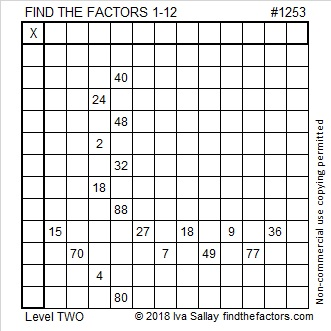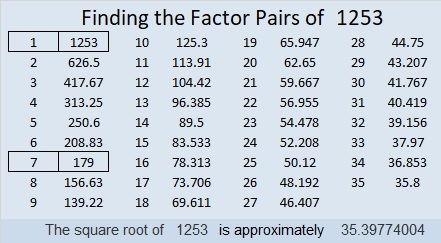# 1253 and Level 2

In what order should the numbers from 1 to 12 be written in the first column and also in the top row so that this puzzle works like a multiplication table?Print the puzzles or type the solution in this excel file: 12 factors 1251-1258

Now I’ll tell you a little bit about the number 1253:

• 1253 is a composite number.
• Prime factorization: 1253 = 7 × 179
• The exponents in the prime factorization are 1 and 1. Adding one to each and multiplying we get (1 + 1)(1 + 1) = 2 × 2 = 4. Therefore 1253 has exactly 4 factors.
• Factors of 1253: 1, 7, 179, 1253
• Factor pairs: 1253 = 1 × 1253 or 7 × 179
• 1253 has no square factors that allow its square root to be simplified. √1253 ≈ 35.397741253 is also the sum of the eleven prime numbers from 89 to 139. Do you know what those prime numbers are?